SIMPLE HARMONIC MOTION

Perhaps the most complicated motion we will study in a high school mechanics course is simple harmonic motion (SHM, for short). Harmonic motion implies that the object is moving through some pattern or path during a given period of time. the object then repeats, or tries to repeat, this path during the next equal period of time. More complicated harmonic oscillators are compound oscillators involving multiple moving objects, or an object with multiple moving parts, such as musical instruments, and damped harmonic oscillators such as automobile springs with shock absorbers attached.
The usual example of a simple harmonic oscillator is the simple pendulum, a mass of significant size attached to a string of negligible mass which in turn is attached to a friction-free support. History shows that Galileo was the first to show that the pendulum kept time as it repeated its motion again and again; the pendulums in question were incense pots swung from chains attached to the ceilings of churches. He measured the time it took for one cycle against his pulse (remember, he was trained as a physician). For a brief discussion of Galileo's role in the pendulum as a timer, go to http://es.rice.edu/ES/humsoc/Galileo/Student_Work/Experiment95/galileo_pendulum.html

A companion example of SHM involves a block of known mass attached to a massless spring whose constant is k and which loses no energy in flexing the spring. It is common for the spring and block to be moving horizontally, so that gravity acts on all parts of the object in the same way.
In either case, it is important to learn where equilibrium is located for the object. It is at this place where Fnet equals zero.When a force of sufficient size is applied to the object, the object is displaced out of equilibrium. When released, some force (gravity for the pendulum, the Hooke's law force for the spring) causes the object to return to the equilibrium position. For this reason, regardless of what other name this force has, it is also called a restoring force. It happens that the greater the displacement from equilibrium, the greater will be the restoring force. That means that the acceleration of the object in question is not uniform; we cannot use page one equations to solve for position or velocity or whatever. Additionally, as the acceleration goes to zero during a cycle, the speed increases to its maximum value. Conversely, as the acceleration vector increases, the velocity vector goes to zero.

Herewith is a derivation of the relationships in questionSimple Harmonic motion

 Consider a block of mass M a resting on a horizontal, friction-free surface. One end of a coil spring is attached to the block; the other end of the spring is attached to the wall. If the block is pulled to one side and released, the force of the spring will be the only force acting on it in the x direction in the object will accelerate in the x- direction. (A net force in the y-direction is 0and these forces will not become a factor in this problem) What we would like to do is displace the block from its equilibrium position and release the block. We would like to know relationships for position-, velocity-, acceleration of this block was a function of time as well as the velocity of the block as a function of position. The law of conservation of energy suggests that the energy stored in the spring at maximum comprression (or extension) manifests itself as potential or kinetic energy whenever the block is somewhere between these two extreme points line 1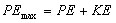Line one is expanded here. "A" in this equation is amplitude, defined as the maximum dslacement. 2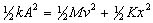Solve line 2 for v (you can do this). This equation yields velocity as a function of position. Give me initial conditions and tell me where the object is and I can determine how fast the block is moving. This is analogous to an earlier kinematics equation See equations, page 1, line 4. 3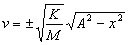One way to define velocity using calculus 4Substitute line 3 into line 4 5Rearrange and prepare to integrate. I can never remember the trick, See http://www.exambot.com/cgi/reference/ show.cgi/math/intc/mint/inv_trig_sub.ref 6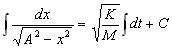ta-dah!!! 7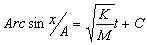We need to establish some initial condition by assigning a value for C. This says we shall start timing the block when it is farthest to the right 8 Let x = A when T = 0 the Let statement is consistent with our initial conditions 9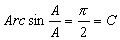The "things" on either side of the equation in line 10 are angles. If two angles are equal then their sines are equal 10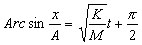line 11 will clean up with an identity. See http://www.exambot.com/cgi/reference/show.cgi/math/trigid.ref 11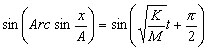Here is an equation for position as a function of time. See page 1, line 3. Take a derivitive wrt time to get line 13 12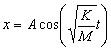A derivative of this line gets to acceleration. See page one line 1 13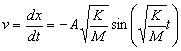Line 14 has no equivalent on page 1. The acceleration is constant there; here it varies witk time. 14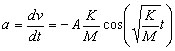O Line 12 tells us that when t = 0, X = A. Let us calculate the elapsed time until the next moment when x = A. Return to line 12. Let x = A 15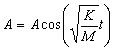In line 15, if x = A, thenmust be an angle whose cosine = 1. Zero radians is a trivial solution (the block has not moved yet). The next angle for whihch this is true is 2 pi radians 16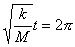Let us solve line 16 for t and while we are at it, let us rename it T. This is called period, the time for one cycle. 17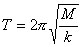Line 18 shows a different arrangement of line 17. The left side is called angular frequency (unit = 1/s). The left side can be substituted for the right hand side in lines 12, 13, & 14 above 18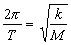Note 1. IUsng the equations in lines 12, 13, 14 above, you can use M and K, or their equivalent 2 pi/ T 19 Note 2. Let' s say an oscillator has an amplitude of 20 cm and a period of 4 s. If the block takes 4 s for one cycle, the it should take two s to go from the far right (x = A) to the far left (x = - A). And if it takes 2 s to go from X = a to x = -A, then it should take 1 s to go from X = +A to x = 0. . 20 Note 3. Calculate x, v and A when T = .5 s. x should be positive (rhs of equlibrium) v should be negative (it is moving left) a should be positive Note 3 stated differrently: if one cycle can be broken into 4 distinct parts, then x is positive in quads 1 & 4 v is positive in quads 3 & 4 a is positive in quads 2 & 3

THE SIMPLE PENDULUM

A simple pendulum consists of a ball attached to one end of a string, the other end is attached to a firm, friction-free point. It is important that the mass of the string is negligible when compared to the mass of the ball. We find that the period of oscillation
T = 2 pi [sq rt (l/g). The carreful reader will note that this equation makes no mention of the mass of the ball or of the size of the initial displacement.

A hallmark of mechanics is the consideration of word problems. Useful relationships are published here in a page called EQUATIONS. The reader should consult this page before attempting the problems assigned.

SIDEBAR
Psst. Got the time? For a discussion of the pendulum as a timer, go to
http://physics.nist.gov/GenInt/Time/revol.html

As the world turns.....For a discussion of the Foucault pendulum, go to
http://www.physics.uoguelph.ca/foucault1.html
http://www.si.edu/resource/faq/nmah/pendulum.htm